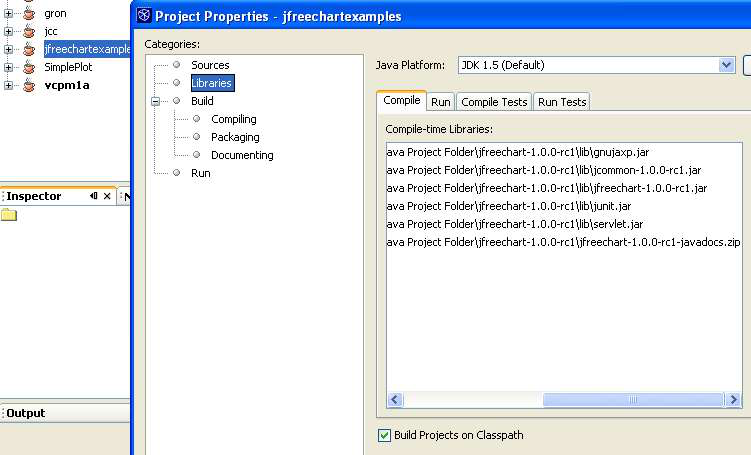Rating : ⭐⭐⭐⭐⭐
Price : \$10.99
Language:EN
Pages: 2

# Defining the axis label cos

*/
//folded code omitted
private void exitMenuItemActionPerformed(java.awt.event.ActionEvent evt) { System.exit(0);
}

/**
* @param args the command line arguments
*/
public static void main(String args[]) {
java.awt.EventQueue.invokeLater(new Runnable() { public void run() {
new example2().setVisible(true);
}
});
}

The cos(x) and cos2(x) are part of the java.Math.* classes which can be imported or explictly coded.

Next, we define two rather than one XYDataset:

CombinedDomainXYPlot parent = new CombinedDomainXYPlot(new NumberAxis("x-angle argument"));

Penultimately, we set up each series to be added:

XYPlot subplot1 = new XYPlot(dataset1,null, new NumberAxis("Cos(x)"),renderer1); NumberAxis axis1 = (NumberAxis)subplot1.getRangeAxis();
axis1.setTickLabelFont(new Font("Monospaced", Font.PLAIN,7));
axis1.setLabelFont(new Font("SansSerif", Font.PLAIN,7));
axis1.setAutoRangeIncludesZero(false);

JFreeChart chart = new JFreeChart("Cos(x) versus x", parent);

BufferedImage image = chart.createBufferedImage(400,500); jLabel1.setIcon(new ImageIcon(image));
this.setSize(500, 600);Illustration 3Figure 3

4
How It Works